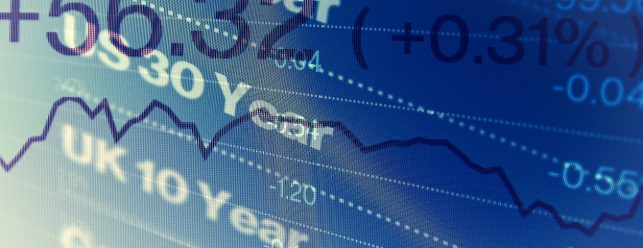This in-person training course provides participants with the essential framework to formulate yield curve trading strategies and presents the analytical tools to apply them in a practical trading context. The course discusses term structure, relative value (RV) methods to detect value along the curve (such as principal component analysis (PCA)), and macro economic impacts on the yield curve.

It also considers trading strategies along a single yield curve and between two yield curves, including swap spreads and basis swaps. Advanced curve fitting methods are presented, enabling delegates to assess the value of individual bonds. Some knowledge of bond market cash and derivative products is assumed. Practical trading examples will be explored in a workshop setting.

Each delegate will be equipped with a PC and Microsoft Excel.

27 - 28 September 2022

Duration:

2 Days

Location:

London, UK – Tower Hotel, London E1

Course Fee:

£1990 + VAT

Register

• Fundamental: Forecasting yields through economic knowledge and expectations
• Statistical: Analysing time series using universal tools, such as mean reversion models
• Adding value with sophisticated models and incorporation of relationships between yield curves

Revision of basic concepts

• Zero, par and forward rates
• Carry and roll-down; The impact of repo rates

Basic fundamental analysis

• Bond yields as a combination of real yields, inflation and a risk premium
• Real yields and economic growth
• Nominal yields and inflation expectations
• Risk premium and fiscal policy
• Supply and demand

• Flatteners/Steepeners, butterflies, condors
• Weighting
• Directionality

Statistical analysis

• Basic: Z-scores
• Advanced: Non-parametric models; Bucketing techniques
• Assessing the impact of targets, stops and scaling on the expected Sharpe ratio
• Finding the optimal execution strategy (e.g., target)

Finding relative value along the yield curve: PCA

• Factor models: Nelson-Siegel and PCA
• Gaining insights into the market mechanisms behind yield curve moves
• Decomposing the yield curve moves into uncorrelated factors
• Identifying and excluding the impact of the direction on yield curve trades: generating alpha
• Hedge ratio calculation
• PCA as a monitor for trades
• PCA as an asset selection tool
• PCA for curve reconstruction and forecasting

Models for the yield curve

• Vasicek model: Modeling the expected path of short term rates
• Shadow rate model: Assessing the impact of negative rates on the shape of the yield curve
• Pitfalls

Fitted curves: Asset selection for yield curve trades

• Different methods for fitting a yield curve through a number of bonds
• Incorporating external information, such as benchmark status
• Analytical value of fitted curves: Constant maturity zero and par rates
• Practical value of fitted curves: Finding the best individual bonds for yield curve trades